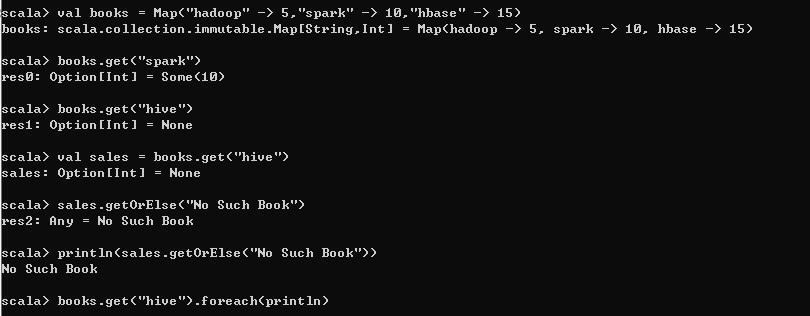# Scala 编程基础笔记﻿

1.模式匹配

a.简单匹配之match语句

﻿

val colorNum = 1 val colorStr = colorNum match{    case 1 => "red"    case 2 => "green"    case 3 => "yellow"    case _ => "Not Allowed"}println(colorStr)

﻿

b.模式匹配的case语句中，还可以使用变量

﻿

val colorNum = 4val colorStr = colorNum match{    case 1 => "red"    case 2 => "green"    case 3 => "yellow"    case unexpected => unexpected + " is Not Allowed"}println(colorStr)

﻿

c.按照类型进行匹配

﻿

for (elem <- List(9,3.18,"Spark","Hadoop",'Hello)){    val type_str = elem match{        case i:Int    => i + " is an int value."        case d:Double => d + " is a double value."        case "Spark"  => "Spark is found."        case s:String => s + " is a string value."        case _        => "This is an unexpected value."    }println(type_str)}

﻿

d.在"=>"左边增加条件表达式

﻿

for (elem <- List(1,2,3,4)){    elem match {        case _ if (elem %2 == 0) => println(elem + " is even.")        case _                   => println(elem + " is odd.")    }}

﻿

e.for循环中的表达式：映射

﻿

for ((k,v) <- 映射) 语句块

﻿

val university = Map("NJU" -> "Nanjing University", "THU" -> "Tsinghua University","PKU"->"Peking University")for ((k,v) <- university) printf("Code is : %s and name is: %s\n",k,v)

﻿

f.case类的匹配：case类是一种特殊的类，它们经过优化以被用于模式匹配

﻿

case class Car(brand:String,price:Int)val Car01 = new Car("BYD",90000)val Car02 = new Car("BMW",100000)val Car03 = new Car("BNZ",110000)for (car <- List(Car01,Car02,Car03)){    car match{        case Car("BYD",90000)  => println("Hello,BYD!")        case Car("BMW",100000) => println("Hello,BMW!")        case Car(brand,price)  => println("Brand: " + brand + ",Price: "+price)    }}

﻿

g.待了解： Option类型，Option类型的子类Some,Any类型，getOrElse方法

﻿

val books = Map("hadoop" -> 5,"spark" -> 10,"hbase" -> 15)books.get("hadoop")books.get("hive")val sales = books.get("hive")sales.getOrElse("No Such Book")println(sales.getOrElse("No Such Book"))books.get("hive").foreach(println)

﻿﻿

2.Scala函数

﻿

a.函数字面量

﻿

val i = 123     //123就是整数字面量val i = 3.14    //3.14就是浮点数字面量val i = true    //true就是布尔型字面量val i = 'A'     //'A'就是字符字面量val i = "Hello" //"Hello"就是字符串字面量

﻿

﻿

def counter(value: Int): Int = { value += 1}

﻿

(Int) => Int

﻿

﻿

(value) => {value += 1} //只有一条语句时，大括号可以省略

﻿

b.Scala定义函数的方式：

﻿

val num: Int = 5

﻿

val counter: (Int) => Int = { (value) => value += 1 }

val counter: Int => Int = { (value) => value += 1 }

﻿

﻿

c.匿名函数、Lambda表达式与闭包

(num: Int) => num * 2

﻿

Lambda表达式的定义公式：

(参数) => 表达式 //如果参数只有一个，参数的圆括号可以省略

﻿

﻿

val NumFunc:Int => Int = (num) => num * 2 println(NumFunc(3))

﻿

"Int => Int",一般可以去掉，因为Scala解释器可以做自动类型推断

*此时需要给num加类型声明

﻿

val NumFunc = (num:Int) => num * 2 println(NumFunc(3))

﻿

d.闭包

﻿

object MyTest{    def main(args: Array[String]): Unit={            def plusStep(step: Int) = (num: Int) => {num + step}        //给step赋值        val myFunc = plusStep(3)        //调用myFunc函数        println(myFunc(10))         }}

﻿

step是一个自由变量，它的值只有在运行的时候才能确定，num的类型是确定的，num的值只有在调用的时候才被赋值。这样的函数，被称为“闭包”，它反映了一个从开放到封闭的过程

﻿

val addMore=(x:Int)=>x>0

val addMore=(x:Int)=>x+more

﻿

more并没有在函数中定义,是一个函数外部的变量,必须在函数外部给出more的值

﻿

var more = 1 val addMore=(x:Int)=>x+moreprintln(addMore(10))

﻿

﻿

﻿

var more = 1 val addMore=(x:Int)=>x+moreprintln(addMore(10))more = 2 println(addMore(10))more = 3 println(addMore(10))

﻿

11

12

13

[Finished in 5.7s]

﻿

e.高阶函数

﻿

[1,3] => 1 + 2 + 3

﻿

def sumFunc(a:Int,b:Int):Int = {    if(a > b) 0 else a + sumFunc(a + 1,b)}

﻿

//定义了一个新的函数sum，以函数f为参数def sum(f: Int => Int, a: Int, b: Int): Int ={   if(a > b) 0 else f(a) + sum(f, a+1, b)}//定义了一个新的函数self，该函数的输入是一个整数x，然后直接输出x自身def self(x: Int): Int = x//重新定义sumInts函数def sumFunc(a: Int, b: Int): Int = sum(self, a, b)

﻿

﻿

﻿

3.占位语法

﻿

val numList = List(-3, -5, 1, 6, 9)numList.filter(x => x > 0 )numList.filter(_ > 0)

"x => x>0" 和 "_ > 0" 等价

﻿

﻿### 郑可夫斯基

Stay hungry ,Stay foolish. 2020.02.15 加入

【微信公众号】背包客Revolution

## 评论